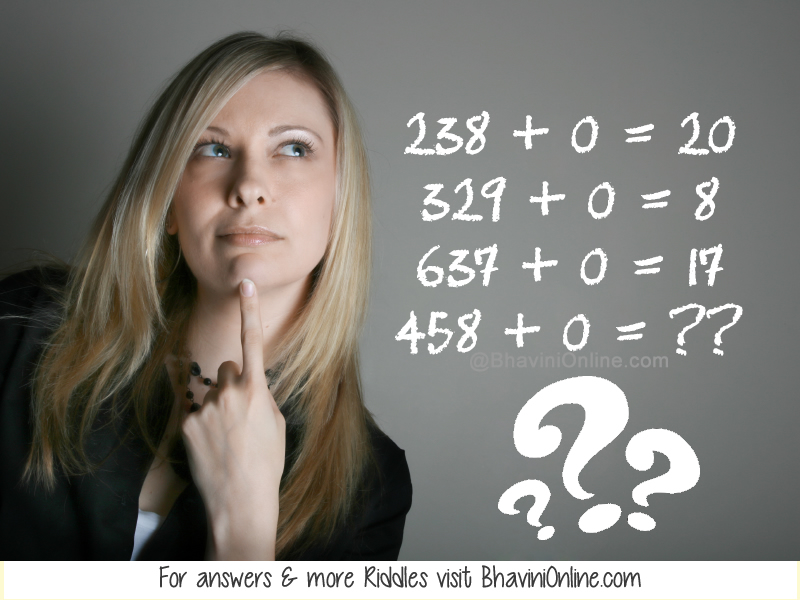# Find the Missing Number: If: 238 + 0 = 20; Then: 458 + 0 = ??

Look at the series of equations given in the riddle and find the logic behind them.

Once you understand how the numbers are related you can find out the missing number in the last equation.

If;

238 + 0 = 20

329 + 0 = 8

637 + 0 = 17

Then;

458 + 0 = ??So were you able to solve the riddle? Leave your answers in the comment section below.

1. Sase says: